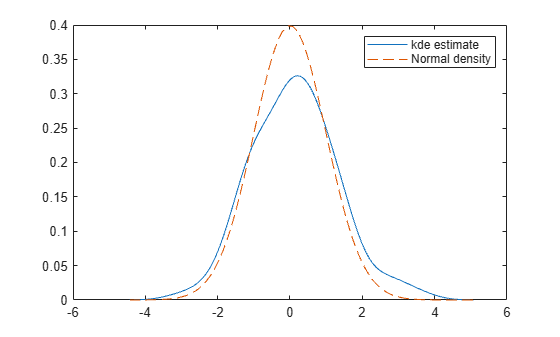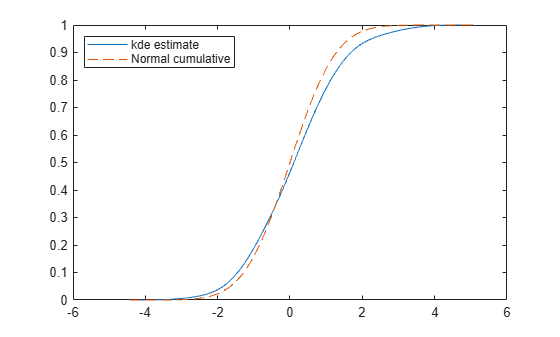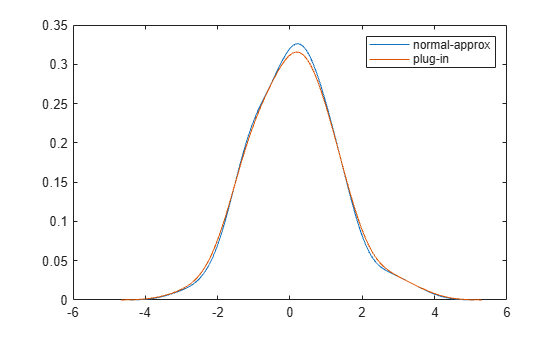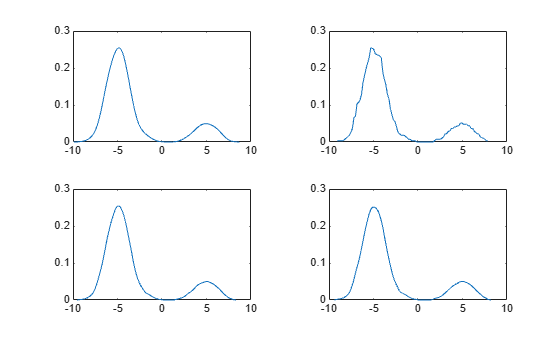# kde

Kernel density estimate for univariate data

Since R2023b

## Syntax

``[f,xf] = kde(a)``
``[f,xf,bw] = kde(a)``
``[___] = kde(a,Name=Value)``

## Description

example

````[f,xf] = kde(a)` estimates a probability density function (pdf) for the univariate data in the vector `a` and returns values `f` of the estimated pdf at the evaluation points `xf`. `kde` uses kernel density estimation to estimate the pdf. See Kernel Distribution for more information.```

example

````[f,xf,bw] = kde(a)` also returns the bandwidth for the kernel smoothing function.```

example

````[___] = kde(a,Name=Value)` specifies options using one or more name-value arguments. For example, `kde(a,ProbabilityFcn="cdf")` estimates the cumulative distribution function (cdf) for `a` instead of the pdf. Use this syntax with any of the output argument combinations in the previous syntaxes.```

## Examples

collapse all

Generate some normally distributed data.

```rng(0,"twister") % For reproducibility a = randn(100,1);```

Estimate the pdf for the sample data.

`[fp,xfp] = kde(a);`

`fp` contains the values for the estimated pdf at the evaluation points in `xfp`.

Estimate the cdf for the sample data.

`[fc,xfc] = kde(a,ProbabilityFcn="cdf");`

`fc` contains the values for the estimated cdf at the evaluation points in `xfc`. `xfc` and `xfp` contain the same evaluation points because they were both calculated with the sample data in `a`.

Evaluate the pdf and cdf for the normal distribution at the evaluation points.

```np = (1/sqrt(2*pi))*exp(-.5*(xfp.^2)); nc = 0.5*(1+erf(xfc/sqrt(2)));```

Plot the estimated pdf with the normal distribution pdf.

```plot(xfp,fp,"-",xfp,np,"--") legend("kde estimate","Normal density")```Plot the estimated pdf with the normal distribution pdf.

```figure plot(xfc,fc,"-",xfc,nc,"--") legend("kde estimate","Normal cumulative",Location="northwest")```The plots show that the estimated pdf and cdf have shapes similar to the pdf and cdf of the standard normal distribution.

Generate some normally distributed data.

```rng(0,"twister") % For reproducibility a = randn(100,1);```

Estimate the pdf for the sample data. By default, `kde` uses the normal-approximation method to calculate the bandwidth for the kernel smoothing function.

`[fn,xfn,bwn] = kde(a);`

`fn` contains the values for the estimated pdf at the evaluation points in `xfn`, and `bwn` is the bandwidth for the kernel smoothing function.

Estimate the pdf using the plug-in method, and display the bandwidth associated with each estimated pdf.

```[p,xp,bwp] = kde(a,Bandwidth="plug-in"); [bwn,bwp]```
```ans = 1×2 0.4958 0.5751 ```

The bandwidth calculated with the normal-approximation method is less than the bandwidth calculated with the plug-in method.

Plot the estimated pdfs.

```plot(xfn,fn) hold on plot(xp,p) legend("normal-approx","plug-in")```The estimated pdfs have shapes typical of a normal distribution. The peak of the pdf corresponding to the normal-approximation method is higher than the peak of the pdf corresponding to the plug-in method.

Generate some bimodal sample data.

```rng(0,"twister") % For reproducibility a = [randn(100,1)-5; randn(20,1)+5];```

Use the default `"normal"` kernel smoothing function to estimate the pdf for the sample data. Use the `"box"`, `"triangle"`, and `"parabolic"` kernel smoothing functions to calculate three more estimates for the pdf.

```[f1,xf1] = kde(a); [f2,xf2] = kde(a,Kernel="box"); [f3,xf3] = kde(a,Kernel="triangle"); [f4,xf4] = kde(a,Kernel="parabolic");```

`xf1`, `xf2`, `xf3`, and `xf4` contain the same evaluation points because they were each calculated with the sample data in `a`. `f1`, `f2`, `f3`, and `f4` contain the values of each estimated pdf at the evaluation points.

Plot the estimated pdfs.

```tiledlayout(2,2) nexttile plot(xf1,f1) % normal nexttile plot(xf2,f2) % box nexttile plot(xf3,f3) % triangle nexttile plot(xf4,f4) % parabolic```The plots show that the four estimated pdfs have similar vertical ranges and two peaks each. The pdf calculated with the `"box"` kernel appears to be the least smooth of the four estimates.

## Input Arguments

collapse all

Sample data used to estimate the probability function, specified as a numeric vector.

Data Types: `single` | `double`

### Name-Value Arguments

Specify optional pairs of arguments as `Name1=Value1,...,NameN=ValueN`, where `Name` is the argument name and `Value` is the corresponding value. Name-value arguments must appear after other arguments, but the order of the pairs does not matter.

Example: `kde(a,Kernel="box",Bandwidth=0.8,Weight=wgt)` specifies a box kernel smoothing function with a bandwidth of `0.8` and vector of observation weights `wgt`.

Bandwidth for the kernel smoothing function, specified as `"normal-approx"`, `"plug-in"`, or a positive scalar.

• When `Bandwidth` is `"normal-approx"`, `kde` uses the normal-approximation method, or Silverman's rule of thumb, to calculate the bandwidth.

• When `Bandwidth` is `"plug-in"`, `kde` uses the improved plug-in method described in  to calculate the bandwidth. The plug-in method is sometimes called the Sheather-Jones method.

• When `Bandwidth` is a positive scalar, its value controls the smoothness of the probability function estimate. As the value increases, the probability function estimate gets smoother.

To see how `Bandwidth` affects the kernel smoothing function, see `Kernel`.

Example: `kde(a,Bandwidth="plug-in")`

Data Types: `single` | `double` | `string` | `char`

Points at which to evaluate the estimated probability function, specified as a numeric vector. By default, `kde` evaluates the estimated probability function at `NumPoints` evenly spaced points that cover the range of the observations in `a`.

If you specify both the `NumPoints` and `EvaluationPoints` name-value arguments, `kde` ignores `NumPoints`.

Example: `kde(a,EvaluationPoints=linspace(0,10,50))`

Data Types: `single` | `double`

Type of kernel smoothing function, specified as a function handle or one of the values in this table.

ValueEquation
`"normal"`${K}_{i}\left(x\right)=\frac{1}{\sqrt{2\pi }}{e}^{\frac{-{d}_{i}^{2}}{2}}$
`"box"`${K}_{i}\left(x\right)=\left\{\begin{array}{c}\frac{1}{2\sqrt{3}},|{d}_{i}|\le \sqrt{3}\\ 0,|{d}_{i}|>\sqrt{3}\end{array}$
`"triangle"`${K}_{i}\left(x\right)=\left\{\begin{array}{c}\frac{1-\frac{|{d}_{i}|}{\sqrt{6}}}{\sqrt{6}},|{d}_{i}|\le \sqrt{6}\\ 0,|{d}_{i}|>\sqrt{6}\end{array}$
`"parabolic"`$\begin{array}{l}{K}_{i,h}\left(x\right)=\mathrm{max}\left(0,\frac{3}{4}u\right),\\ u=\frac{1-\frac{{z}^{2}}{5}}{\sqrt{5}},\\ z=\mathrm{max}\left(-\sqrt{5},\mathrm{min}\left({d}_{i},\sqrt{5}\right)\right)\end{array}$

In the table, ${d}_{i}=\frac{x-{a}_{i}}{h}$, h is the bandwidth specified in the `Bandwidth` name-value argument, and `ai` is the element at position `i` in `a`. A parabolic kernel smoothing function is sometimes called an epanechnikov smoothing function.

If you specify `Kernel` as a function handle, the function must accept a matrix or column vector of arbitrary length as its only input argument and return a nonnegative matrix or vector of the same size.

For more information about how `kde` uses the kernel smoothing function to estimate the probability function, see Kernel Distribution.

Example: `kde(a,Kernel="parabolic")`

Data Types: `string` | `char` | `function_handle`

Number of evaluation points for the estimated probability function, specified as a positive integer scalar. By default, `NumPoints = max(100,u)`, where `u` is the square root of the number of elements in `a`, rounded to the nearest integer.

If you specify both the `NumPoints` and `EvaluationPoints` name-value arguments, `kde` ignores `NumPoints`.

Example: `kde(a,NumPoints=100)`

Data Types: `single` | `double`

Probability function to estimate, specified as `"pdf"` or `"cdf"`. When `ProbabilityFcn` is `"pdf"`, `kde` estimates a probability density function. To estimate a cumulative distribution function, specify `ProbabilityFcn` as `"cdf"`.

Example: `kde(a,ProbabilityFcn="cdf")`

Interval for the sample data, specified as a two-element numeric vector, `"unbounded"`, `"positive"`, `"nonnegative"`, or `"negative"`. The elements of `a` must be in the interval specified by `Support`. The estimated probability function evaluates to `0` outside of the interval.

If you specify `Support` as a two-element vector ```[L U]``` or `[L;U]`, `L` must be greater than `max(a)` and `U` must be less than `min(a)`. The interval is open with lower bound `L` and upper bound `U`.

If you specify `Support` as a string, the sample data exists inside an interval described in this table.

ValueSupport
`"unbounded"`$\left(-Inf,Inf\right)$
`"positive"`$\left(0,Inf\right)$
`"nonnegative"`$\left[0,Inf\right)$
`"negative"`$\left(-Inf,0\right)$

Example: `kde(a,Support="nonnegative")`

Data Types: `single` | `double` | `string` | `char`

Observation weights, specified as a nonnegative vector. By default, `kde` weights all observations in `a` equally. For more information about how `kde` uses weights to estimate the probability function, see Kernel Distribution.

Data Types: `single` | `double`

## Output Arguments

collapse all

Estimated function values, returned as a numeric vector. The length of `f` is equal to the number of evaluation points in `xf`.

Evaluation points, returned as a numeric vector. `xf` has the same size as the `EvaluationPoints` name-value argument, if `EvaluationPoints` is specified. Otherwise, the size of `xf` is given by the `NumPoints` name-value argument.

Bandwidth for the kernel smoothing function, returned as a positive scalar. You can use the `Bandwidth` name-value argument to specify the value for `bw` or the method for calculating `bw`.

collapse all

### Kernel Distribution

A kernel distribution is a nonparametric representation of a probability density function (pdf) of a random variable. You can use a kernel distribution when a parametric distribution cannot properly describe the data or when you want to avoid making assumptions about the distribution of the data. A kernel distribution is defined by a smoothing function and a bandwidth value, which control the smoothness of the resulting density curve.

The kernel estimator is an estimated probability function for a random variable. For any real values of x, the kernel estimator for the pdf is given by

`${\stackrel{^}{f}}_{h}\left(x\right)=\frac{1}{nh}\sum _{i=1}^{n}{w}_{i}K\left(\frac{x-{x}_{i}}{h}\right)\text{\hspace{0.17em}},$`

where the xi values are random samples from an unknown distribution, wi values are their corresponding weights, n is the sample size, $K$ is the kernel smoothing function, and h is the bandwidth.

For any real values of x, the kernel estimator for the cumulative distribution function (cdf) is given by

`${\stackrel{^}{F}}_{h}\left(x\right)={\int }_{-\infty }^{x}{\stackrel{^}{f}}_{h}\left(t\right)dt=\frac{1}{nh}\sum _{i=1}^{n}{w}_{i}G\left(\frac{x-{x}_{i}}{h}\right)\text{\hspace{0.17em}},$`

where $G\left(x\right)={\int }_{-\infty }^{x}K\left(t\right)dt$.

For more details, see Kernel Distribution (Statistics and Machine Learning Toolbox).

 Botev, Z. I., J. F. Grotowski, and D. P. Kroese. "Kernel Density Estimation via Diffusion." The Annals of Statistics, vol. 38, no. 5 (October 1, 2010). https://projecteuclid.org/journals/annals-of-statistics/volume-38/issue-5/Kernel-density-estimation-via-diffusion/10.1214/10-AOS799.full

 Bowman, A. W., and A. Azzalini. "Applied Smoothing Techniques for Data Analysis." New York: Oxford University Press Inc., 1997.

 Hill, P. D. "Kernel estimation of a distribution function." Communications in Statistics - Theory and Methods. 14, no. 3(January 1985): 605–620.

 Jones, M. C. "Simple boundary correction for kernel density estimation." Statistics and Computing. no. 3(September 1993): 135–146.

 Silverman, B. W. "Density Estimation for Statistics and Data Analysis." Chapman & Hall/CRC, 1986.